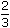# Percentage Aptitude Questions and Answers mcq

1. If 20% of a = b, then b% of 20 is the same as:

•  4% of a
•  2% of a
• 40% of a
• 10% of a
2. In a certain school, 20% of students are below 8 years of age. The number of students above 8 years of age isof the number of students of 8 years of age which is 48. What is the total number of students in the school?
• 100
• 198
• 150
• 50
3 . Two numbers A and B are such that the sum of 5% of A and 4% of B is two-third of the sum of 6% of A and 8% of B. Find the ratio of A : B.
• 4:3
• 4:5
• 6:5
• 3:2

 4 . A student multiplied a number by 3 instead of 5 . 5 3
What is the percentage error in the calculation?
• 64%
• 56%
• 45%
• 12%
5 . In an election between two candidates, one got 55% of the total valid votes, 20% of the votes were invalid. If the total number of votes was 7500, the number of valid votes that the other candidate got, was:
• 2700
• 5600
• 4500
• 6700
6 . A batsman scored 110 runs which included 3 boundaries and 8 sixes. What percent of his total score did he make by running between the wickets?
• 500/11
• 459/11
• 45/10
• 23/10

 7  . Two students appeared at an examination. One of them secured 9 marks more than the other and his marks was 56% of the sum of their marks. The marks obtained by them are: 43,33 45,67 12,11 45,89 8. A fruit seller had some apples. He sells 40% apples and still has 420 apples. Originally, he had: 700  596 598 600 9 . What percentage of numbers from 1 to 70 have 1 or 9 in the unit's digit? 20 12 45 90 10 . Three candidates contested an election and received 1136, 7636 and 11628 votes respectively. What percentage of the total votes did the winning candidate get? 57% 34% 67% 78%